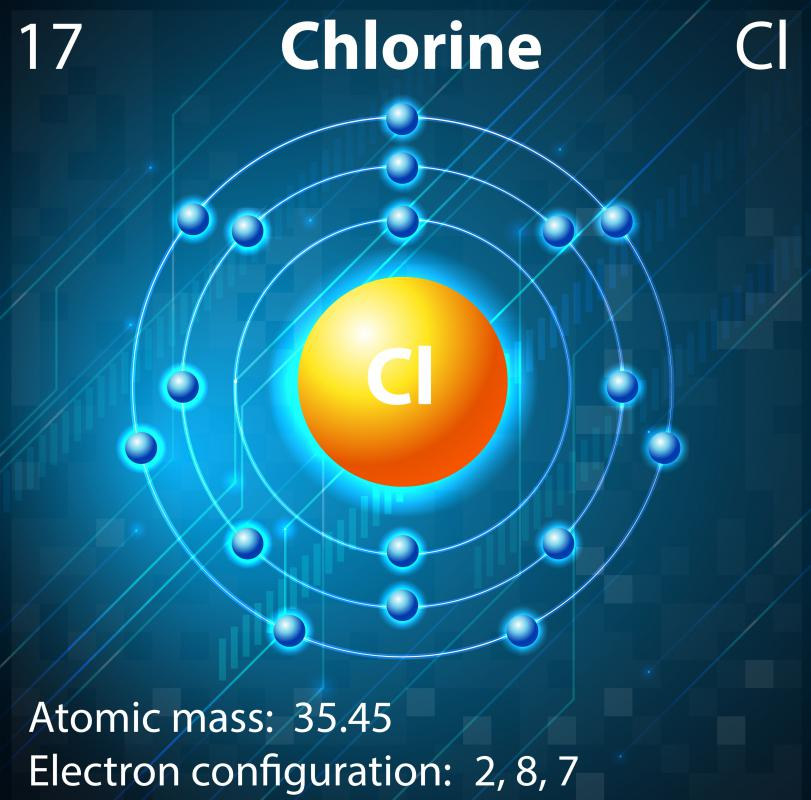# What Is Redox Potential?

Phil Riddel
Phil RiddelChlorine is a strong oxidizing agent and causes oxidation by accepting electrons from a compound.

Redox potential, also known as standard electrode potential, is a measure of how easily a substance loses or gains electrons in a reduction-oxidation — or “redox” — reaction, a chemical reaction where one reactant is reduced and the other oxidized. Electrons move from the reducing agent to the oxidizing agent. This can be expressed as an electrical potential between the two and measured in volts. A negative value indicates a reducing agent, with a tendency to lose electrons, and a positive value indicates an oxidizing agent, with a tendency to gain electrons. The redox potential is sometimes denoted by E°.

In practical terms, redox potential can only be measured for pairs of substances, and whether electrons flow from or to a given chemical depends on the other member of the pair. It is therefore a relative, rather than an absolute, value. In order to establish standard values for elements, compounds and ions, redox potential measurement is performed against a “standard” of hydrogen, which is taken to have an E° of zero, so all such values are relative to hydrogen.

To determine the E° for a given substance, an electrochemical cell consisting of two half-cells is constructed. One consists of H+ ions and neutral hydrogen in equilibrium and is known as a standard hydrogen electrode. The other contains the substance to be tested, again with ionic and neutral forms in equilibrium. The two are linked by a salt bridge containing a suitable electrolyte and the cell is connected to a voltmeter, completing the circuit. Where there is a difference in redox potential, electrons will try to flow one way or the other, creating a potential difference that is measured by the voltmeter — in this case, a high-resistance voltmeter is used in order to prevent any actual flow of current, as this would reduce the potential.

If electrons flow from the hydrogen half-cell to the other, the substance has a positive redox potential and is, in this context, an oxidizing agent. If electrons flow the other way, the E° is negative, indicating a reducing agent. The terms “oxidizing” and “reducing” as used here are relative to hydrogen — the chemical being tested may behave differently relative to a different agent. For example, in this scenario hydrogen is acting as a reducing or an oxidizing agent, depending on what is in the other half cell.

In this way a standard redox potential table has been compiled, showing the E° values for various “half-reactions” involving the addition of electrons to a given agent, as would be the case in one half of a redox reaction. For example, the redox potential for a lithium ion gaining an electron is shown as the half-reaction: Li+ + e- -> Li with an E° value of -3.05 volts, indicating that lithium is a strong reducing agent. The value for the formation of chloride ions by the addition of electrons to chlorine is shown as Cl2 + 2e- ->2Cl- with an E° of +1.36 volts, so chlorine is an oxidizing agent. By knowing the redox potentials of two substances it is possible to predict whether a redox reaction between them is theoretically possible.

## You might also Like